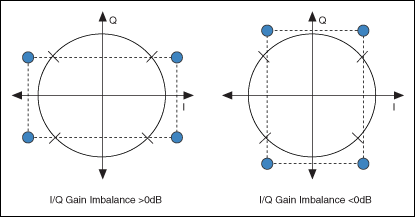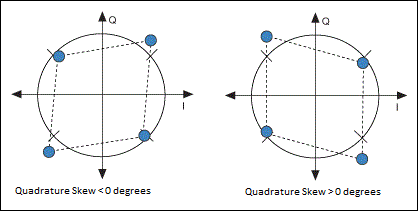# I/Q Impairments

NI-RFmx Demod 3.0 Help

Edition Date: January 2019

Part Number: 374641K-01

»View Product InfoDownload Help (Windows Only)

In a quadrature modulated (QM) system, the ideal QM waveform is the sum of the I and Q components of the signal, as shown in the following equation.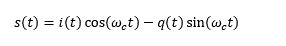where, i(t) is the baseband I waveform q(t)is the baseband Q waveform

The practical QM waveform differs from the ideal QM waveform. A generalized adjusted QM waveform can be expressed as shown in the following equation.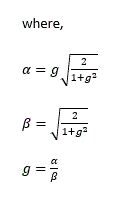and, g is the gain imbalance φ is the quadrature skew ΔI is the in-phase DC offset ΔQ is the quadrature-phase DC Offset

The vector sum of the in-phase DC offset and the quadrature –phase DC offset results in origin offset.

## Origin offset

The origin offset, measured in dB, is the distance between the ideal constellation center to the actual constellation center, as shown in the following figure.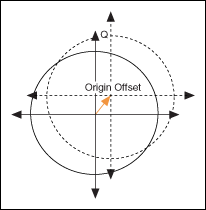Before measuring EVM, the symbols are corrected for origin offset.

## I/Q Gain Imbalance

I/Q gain imbalance refers to the difference in scaling between the I and Q components of I/Q data.

When expressed in dB, I/Q gain imbalance can be either positive or negative, with the sign indicating which component has been impaired, as denoted in the following figure.